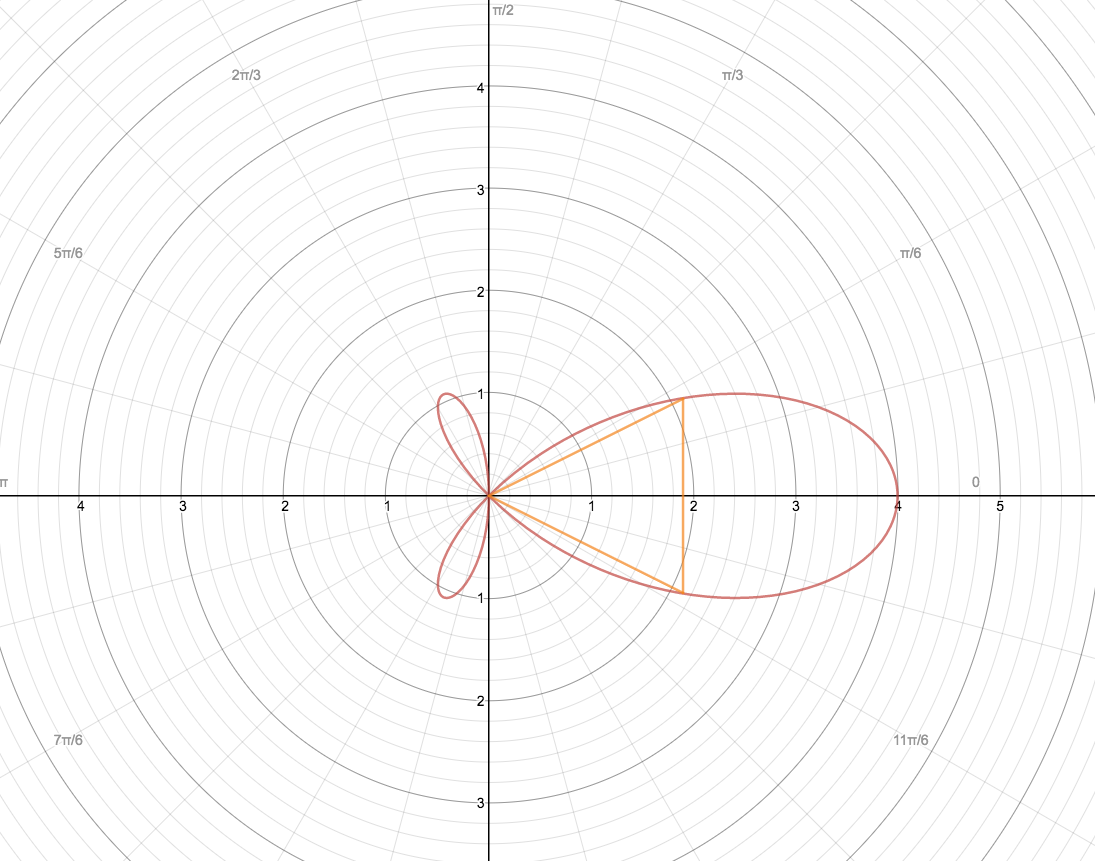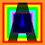# A Question about a recent Problem of the Week

I was trying out the problem of the week about the polar graph $r=\frac{\sin\left(4\theta\right)}{\sin\left(\theta\right)}$ and its volume of revolution about the x-axis. My method is quite different from all of the solutions that have been posted, and so I'm having trouble figuring out why it doesn't work. My goal was to solve the problem without converting to rectangular coordinates. As such, I represented the area using a bunch of cones with top points at the origin and centered along the x-axis. The lateral surface area of these cones would then be integrated to obtain the volume, much in the same way that the method of cylindrical shells works. (See the diagram for a quick image -- the rotation of the triangle around the x-axis is one of the cones whose lateral surface area is being calculated and integrated.) We exclude the area of the base of each cone because the bases alone are the disks that would be used in disks/washers, and so if we included those as well we'd be covering the volume more than once.This method has some restrictions that I figured out, but the graph in the problem shouldn't pose any difficulties for it. At least intuitively, just by visualizing the problem and the filling of the volume with the lateral surface areas of the cones, I see nothing wrong with this method.

The lateral surface area of a cone with lateral height (height from edge of the bottom to the point on the top) $l$ and base radius $R$ is $\pi R l$. At some angle $\theta$, the lateral height of the cone on the polar graph in question is just $r=\frac{\sin\left(4\theta\right)}{\sin\left(\theta\right)}$. The radius of this cone is equal to $r \sin (\theta)$. Therefore the lateral surface area is $\pi r \sin (\theta) \frac{\sin\left(4\theta\right)}{\sin\left(\theta\right)} =\pi \frac{\sin^2\left(4\theta\right)}{\sin\left(\theta\right)}$.

Therefore, the volume of the part in the first quadrant when rotated about the x-axis is equal to $\pi\int_0^{\frac{\pi}{4}}\frac{\sin^2\left(4\theta\right)}{\sin\left(\theta\right)}d\theta$. According to WolframAlpha, this has a precise value of $\frac{176\pi}{105}-\frac{32\pi\sqrt{2}}{105}$. The volume of the remaining part in the second quadrant is $\pi\int_{\frac{\pi}{2}}^{\frac{3\pi}{4}}\frac{\sin^2\left(4\theta\right)}{\sin\left(\theta\right)}d\theta$. This has an exact value of $\frac{32\pi\sqrt{2}}{105}$. The volume of the entire revolution is the sum of these two values, which is simply $\frac{176\pi}{105}$. But this is far smaller than the correct solution of $\frac 8 3 \pi$.

Where did this method go wrong? I'm having trouble seeing anything, and I'm pretty sure the mechanics of the integrals are correct, so it's probably something with the method that I'm not seeing.Note by Aram Lindroth
3 years, 1 month ago

This discussion board is a place to discuss our Daily Challenges and the math and science related to those challenges. Explanations are more than just a solution — they should explain the steps and thinking strategies that you used to obtain the solution. Comments should further the discussion of math and science.

When posting on Brilliant:

• Use the emojis to react to an explanation, whether you're congratulating a job well done , or just really confused .
• Ask specific questions about the challenge or the steps in somebody's explanation. Well-posed questions can add a lot to the discussion, but posting "I don't understand!" doesn't help anyone.
• Try to contribute something new to the discussion, whether it is an extension, generalization or other idea related to the challenge.

MarkdownAppears as
*italics* or _italics_ italics
**bold** or __bold__ bold
- bulleted- list
• bulleted
• list
1. numbered2. list
1. numbered
2. list
Note: you must add a full line of space before and after lists for them to show up correctly
paragraph 1paragraph 2

paragraph 1

paragraph 2

[example link](https://brilliant.org)example link
> This is a quote
This is a quote
    # I indented these lines
# 4 spaces, and now they show
# up as a code block.

print "hello world"
# I indented these lines
# 4 spaces, and now they show
# up as a code block.

print "hello world"
MathAppears as
Remember to wrap math in $$ ... $$ or $ ... $ to ensure proper formatting.
2 \times 3 $2 \times 3$
2^{34} $2^{34}$
a_{i-1} $a_{i-1}$
\frac{2}{3} $\frac{2}{3}$
\sqrt{2} $\sqrt{2}$
\sum_{i=1}^3 $\sum_{i=1}^3$
\sin \theta $\sin \theta$
\boxed{123} $\boxed{123}$

Sort by:

OK, I think I see what's wrong here: the infinitesimally small conical shells can't just be multiplied by $d\theta$ in order to obtain the change in volume $dV$. They have a conical shape with a nonuniform thickness, so I need to take that into account. I'm working out the details now, but the result involves much more complicated integrands. that accurately represent the stranger shape of the conical shells.

- 3 years, 1 month ago

I tried this approach at first as well but I realized it wasn't working because the answer I kept getting was intuitively too small. I tried the same thing revolving the circle $r=2cos(\theta)$ since that has a known volume, but I couldn't get it to work.

- 3 years, 1 month ago